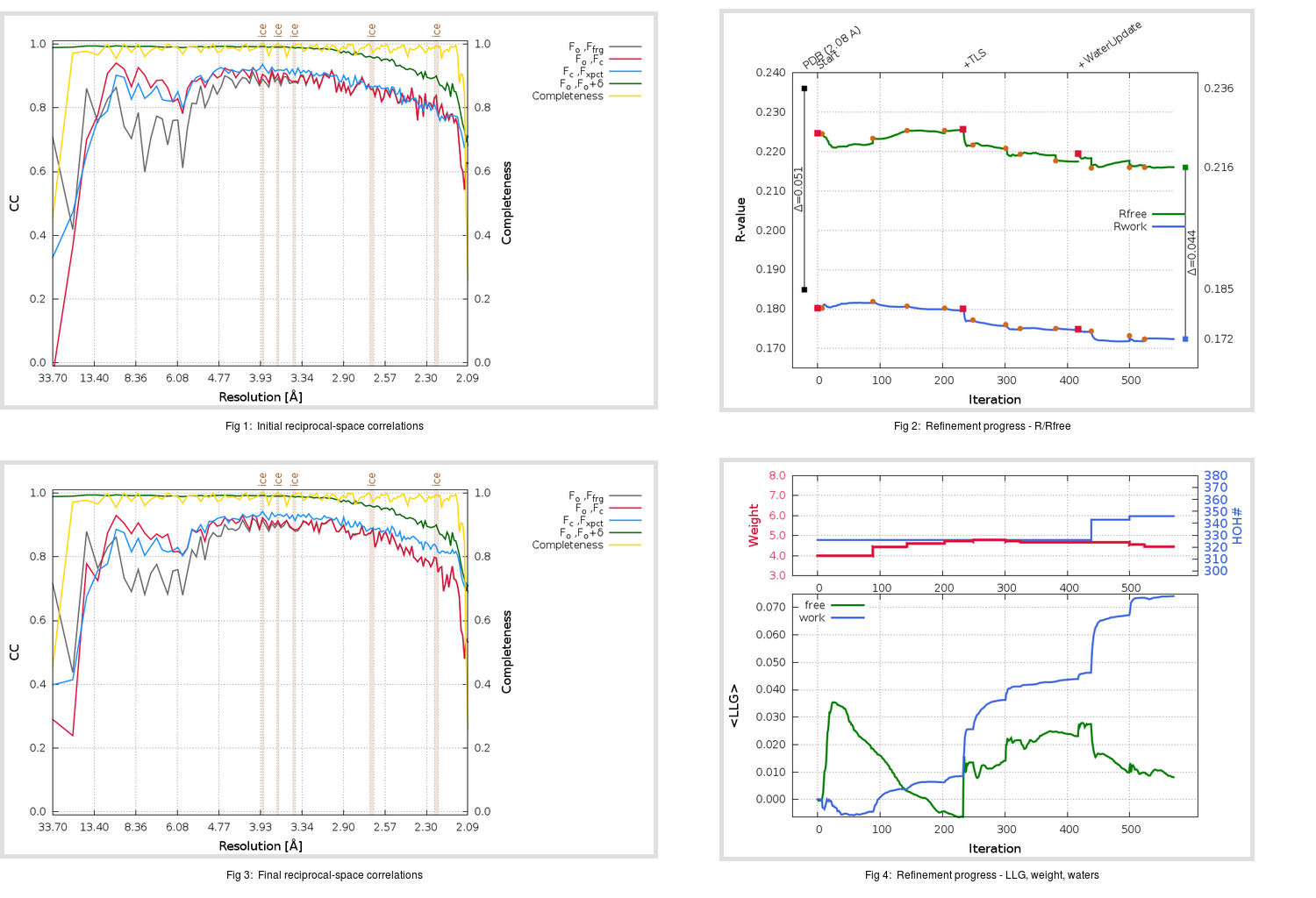Content:

```    Diffraction limits & principal axes of ellipsoid fitted to diffraction cut-off surface:
2.060         0.9841   0.0000   0.1776       0.999 a* - 0.037 c*
2.039         0.0000   1.0000   0.0000       b*
2.002        -0.1776   0.0000   0.9841      -0.339 a* + 0.941 c*
```

## Deposited

` `
 Date deposited Date data collection Resolution R, Rfree 20110822 20100515 2.08 0.1830 0.2360

Molprobity (CCP4 7.0 version) summary:

```Ramachandran outliers =   0.33 %
favored =  96.38 %
Rotamer outliers      =   0.75 %
C-beta deviations     =     1
Clashscore            =   6.22
RMS(bonds)            =   0.0153
RMS(angles)           =   1.41
MolProbity score      =   1.58
Resolution            =   2.08
R-work                =   0.1830
R-free                =   0.2360
```

```Number of waters      =   326

<B> (all atoms) =   27.10 ( sd =    9.64 ) for       2760 non-hydrogen atoms
<B>   (protein) =   26.01 ( sd =    8.68 ) for       2380 non-hydrogen atoms
<B>     (water) =   31.14 ( sd =    9.81 ) for        326 non-hydrogen atoms
<B>    (others) =   50.35 ( sd =   12.22 ) for         54 non-hydrogen atoms

B min/max       (all non-hydrogen atoms) =    6.05 /   69.79
B min/max   (protein non-hydrogen atoms) =    6.05 /   65.54
B min/max     (water non-hydrogen atoms) =    9.50 /   58.56
B min/max     (other non-hydrogen atoms) =   28.32 /   69.79
```

## BUSTER (re-)refinement

` `

Molprobity (CCP4 7.0 version) summary:

```Ramachandran outliers =   0.33 %
favored =  97.70 %
Rotamer outliers      =   1.51 %
C-beta deviations     =     0
Clashscore            =   3.52
RMS(bonds)            =   0.0116
RMS(angles)           =   1.58
MolProbity score      =   1.35
Resolution            =   2.08
R-work                =   0.1725
R-free                =   0.2161
```

```Number of waters      =   346

<B> (all atoms) =   30.61 ( sd =   10.66 ) for       2780 non-hydrogen atoms
<B>   (protein) =   28.58 ( sd =    9.15 ) for       2380 non-hydrogen atoms
<B>     (water) =   41.65 ( sd =   11.10 ) for        346 non-hydrogen atoms
<B>    (others) =   49.33 ( sd =    6.97 ) for         54 non-hydrogen atoms

B min/max       (all non-hydrogen atoms) =   12.57 /   80.73
B min/max   (protein non-hydrogen atoms) =   12.57 /   80.73
B min/max     (water non-hydrogen atoms) =   15.42 /   72.56
B min/max     (other non-hydrogen atoms) =   37.09 /   59.72
```

Refinement progression:Results:

` `
 File Remark 3TIU_aB_refine.01_03_refine.pdb.gz exact refinement commands are in header 3TIU_aB_refine.01_03_refine.mtz.gz including original deposited data and several re-refinement map coefficients 3TIU_aB_refine.01_03_BUSTER_model.cif.gz including any non-standard compound restraints 3TIU_aB_refine.01_03_BUSTER_refln.cif.gz